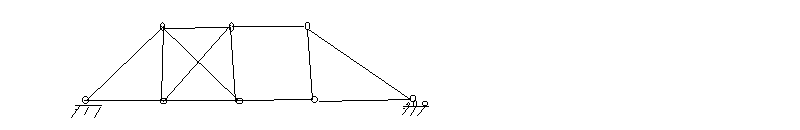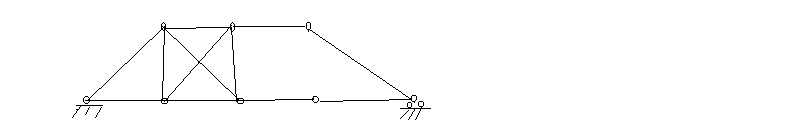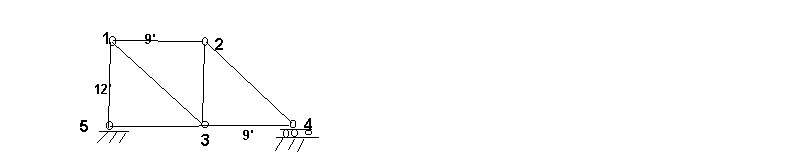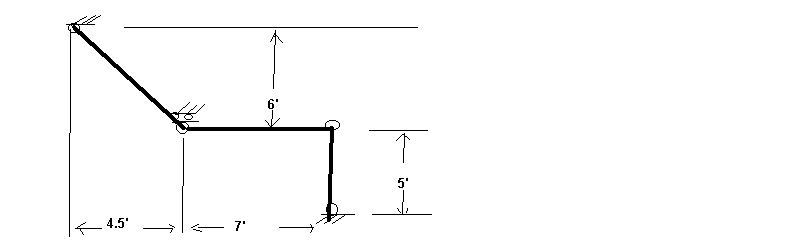Week #1       Project #4          Truss Analysis by the Method of Joints

Introduction In Civil Engineering, trusses are a fundamental component of common structures such as buildings and bridges.Recent studies by the federal government show that a very high number of bridges in the US are in a substandard, and in some cases dangerous, state. For example, 50% of those in Massachusetts are, while 60% in New York state are (in some cases they are falling apart while in others they are simply too narrow). As many US bridges were built in the 50s and 60s and were intended for 30 years life, bridge repair and construction is an important area.In this project, you are introduced to basic notions of truss analysis, as well as how solutions of systems of linear equations relates to the analysis.

background: You should be able to use free body diagrams and solve systems of equations. Feel free to use software such as Maple to make the work quicker!

Trusses A truss consists of a number of bars which are connected by pins. We assume the truss lies in a plane so all considerations are two dimensional. A simple example is below:Static

Terminology and notation:

Note that two joints are fixed. In what follows, j is the number of joints, so 8 in this case. The external force acting on each joint has two components, horizontal and vertical. Thus there are 2j external forces and reactions to those forces. Let NP denote the total number of external forces on joints and NR denote the total number of reactions. Then

(1)                NP + NR = 2j

Further, let NF be the number of bars, 13 in this case.

The study of static trusses is interested, for a given situation, whether it is algebraically possible for the truss to be in static equilibrium. This is equivalent to, in the realm of linear algebra, to seeing if a certain set of equations for static equilibrium of forces has a solution (it is a nonhomogeneous linear system, so it may or may not have one).

The study of static trusses is also interested in whether or not the system of equations contains a different number of equations than unknowns or the same number. If it has a different number, it said to be statically indeterminate. Otherwise it is said to be statically determinate. In other words, it is desirable to have the same number of equations as unknowns – that is what static indeterminacy amounts to.

The System of Equations of a Static Truss

If there are j pins, then for each one, the sum of the forces is 0. Since there are 2 components of force for each pin, this means there are 2j equations for force components. These are independent equations.

Assume each pin has an external force Fi acting on it. Fi is a vector with two components, and there are p such vectors, so i takes on values from 1 to p.

Assume the internal forces between connected pins are Pi. Pi is also a vector with two components and there is one P for every bar between pins (so i takes on values from 1 to NF)

_______________

Problem Show that the definition of static determinacy requires that NF = NP (hint: start with equation (1)

Problem 2 Why do you think it is possible for a truss to be statically determinate yet still be statically unstable? In your discussion, consider the following truss:Problem 3 Why is the following truss statically indeterminate?(in the above truss, the rightmost pin is free to move horizontally, while the leftmost pin can not move at all)

The system of equations, continued:

To make all this more explicit, we develop the system of equations for the truss below:We first note that it is statically determinate because NP = NF = 7 (the reader is urged to check this). By using the free-body diagrams for each of the 5 joints and taking into account that the lower left hand joint is fixed while the lower right hand joint can only move horizontally, we get 7 equations:

P1 = -1.0 F1 - .6 F6            (pin 1, horizontal component)

P2 = 1.0 F4 + .8 F6             (pin 1, vertical component)

P3 = 1.0 F1 - .6 F7              (pin 2, horizontal component)

P4 = 1.0 F5 + .8 F7             (pin 2, vertical)

P5 = 1.0 F2 - 1.0 F3 + .6F6       (pin 3, horizontal component)

P6 = -1.0 F5 - .8 F6               (pin 3, vertical)

P7 = 1.0 F3 + .6 F7            (pin 4, horizontal component)

show that this system has a solution for F and that it is unique.

In matrix form, this system may be written as    P = A F  where A is called the statics matrix of the truss. The condition of static determinacy means that A will be a square matrix.

Problems For each statically determinate truss below,

1. decide if it is statically determinate
2. if it is statically determinate, set up the equations F = AP
3. solve the equations and decide if the truss is stable

Truss 1:Truss 2:Final problem: from linear algebra, we know that one can begin with more equations than unknowns and still have a unique solution (in the RREF, the rank would have to equal the number of unknowns) Discuss the requirement of static determinancy in light of this.